# ACT Math : How to find the length of the hypotenuse of an acute / obtuse triangle

## Example Questions

### Example Question #1 : How To Find The Length Of The Hypotenuse Of An Acute / Obtuse Triangle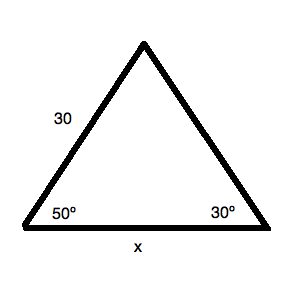What is the value ofin the triangle above? Round to the nearest hundredth.Cannot be calculated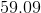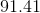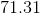Explanation:

Begin by filling in the missing angle for your triangle. Since a triangle has a total ofdegrees, you know that the missing angle is: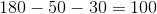Draw out the figure: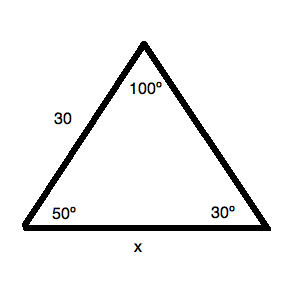Now, to solve this, you will need some trigonometry. Use the Law of Sines to calculate the value: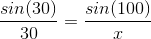Solving for, you get: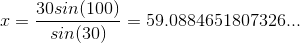Rounding, this is.